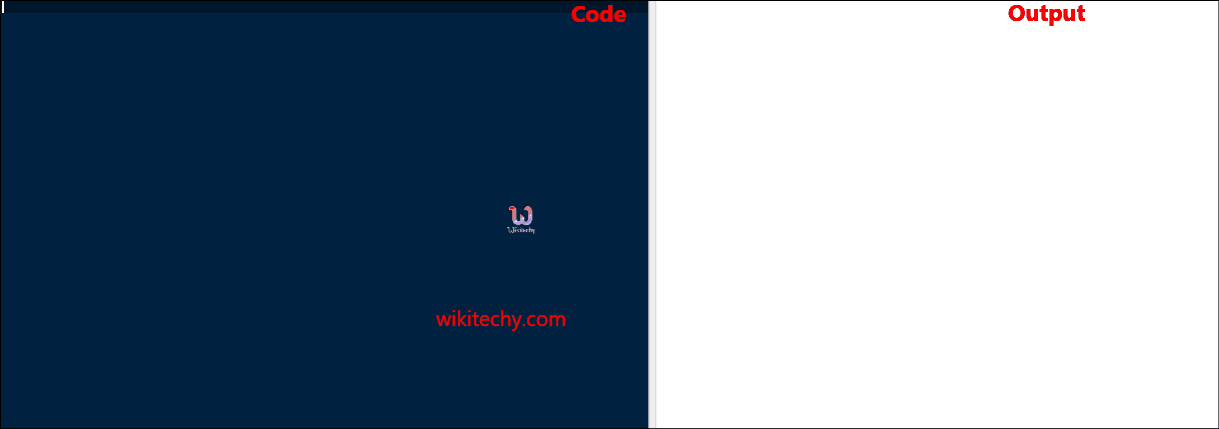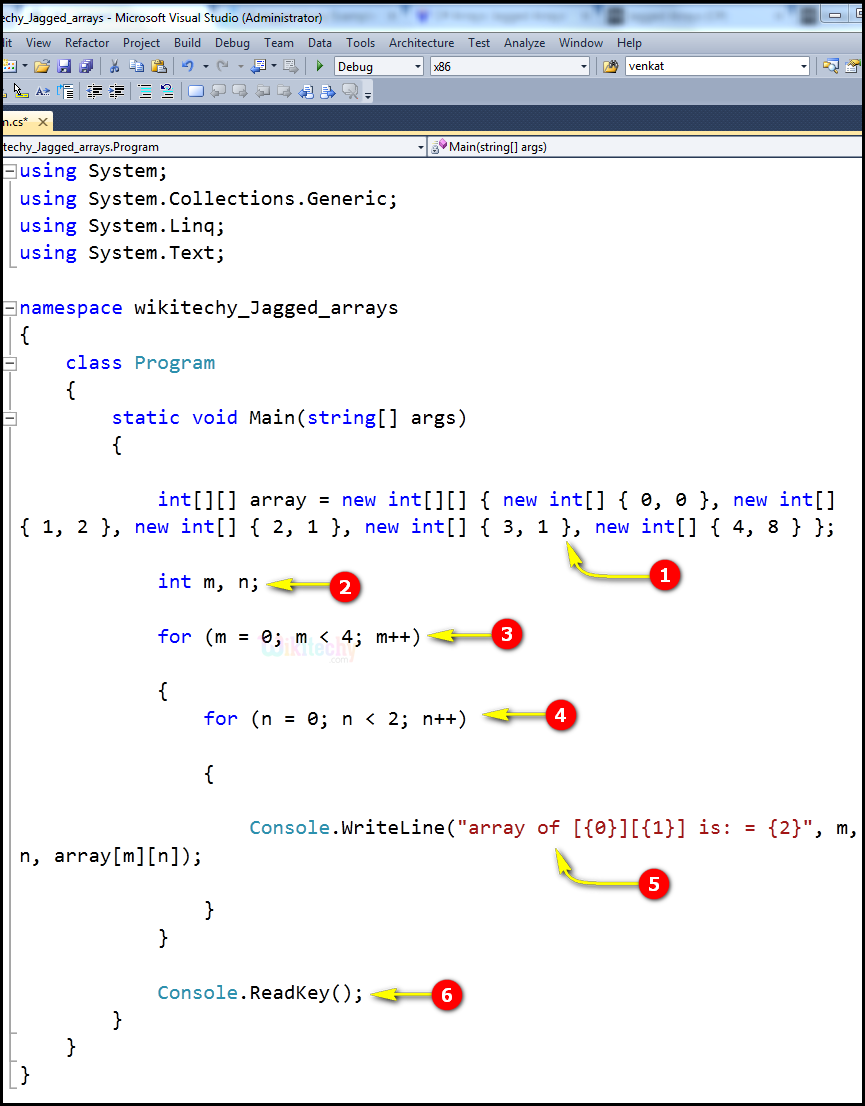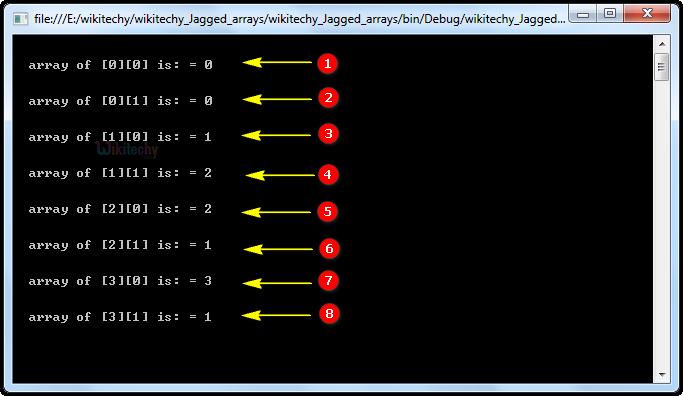# C# Jagged array - c# - c# tutorial - c# netC# Jagged Array

## What is jagged array in C# ?

• A jagged array is an array whose elements are arrays.
• The elements of a jagged array can be of different dimensions and sizes.
• A jagged array is sometimes called an "array-of-arrays".## Syntax Explanation:

• datatype:
• Datatype is used to specify the type of elements in the array.
• [] []:
• In jagged arrays, the first square bracket can be used as its own one-dimensional array or as a multi-dimensional array.

## Code Explanation:1. We declare and initialize a numerical array. And we declare jagged array of 5 array of integers.
2. Here int m, n ; is an integer variables.
3. for (m = 0; m< 4; m++) specifies integer m = 0, which means the expression, (m < 4), is true. Therefore, the statement is executed, and m gets incremented by 1 and becomes less than 3.
4. for (n = 0; n< 2; n++) specifies integer n = 0, which means the expression, (n < 2), is true. Therefore, the statement is executed, and n gets incremented by 1 and becomes less than 2.
5. Here we print the value of "m, n, array[m][n]" using the "array of" statement.
6. Obtains the next character or function key pressed by the user. The pressed key is displayed in the console window.

## Sample C# examples - Output :1. Here in this output the jagged array elements  is represented as a console statement. The array of elements  value as "0"
2. Here in this output the jagged array elements  is represented as a console statement. The array of elements  value as "0"
3. Here in this output the jagged array elements  is represented as a console statement. The array of elements  value as "1"
4. Here in this output the jagged array elements  is represented as a console statement. The array of elements  value as "2"
5. Here in this output the jagged array elements  is represented as a console statement. The array of elements  value as "2"
6. Here in this output the jagged array elements  is represented as a console statement. The array of elements  value as "1"
7. Here in this output the jagged array elements  is represented as a console statement. The array of elements  value as "3"
8. Here in this output the jagged array elements  is represented as a console statement. The array of elements  value as "1"